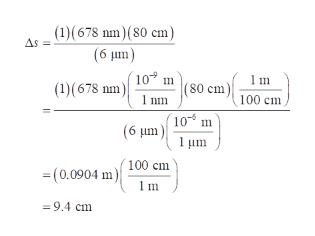Question
41 views

The figure shows the interference pattern that appears on a distant screen when coherent light is incident on a mask with two identical, very narrow slits. Points P and Q are maxima; Point R is a minimum. The wavelength of the light that created the interference pattern is λ=678nm, the two slites are separated by rm d=6 μm, and the distance from the slits to the center of the screen is L=80cm . The difference in path length at a point on the screen is Δs=|s1−s2|, where s1s1 and s2s2 are the distances from each slit to the point.

What is ΔsΔs (in nm) at Point P?

What is ΔsΔs (in nm) at Point Q?

What is ΔsΔs (in nm) at Point R?

check_circle

Step 1

The point P is at central maxima. Therefore, the path length “delta s” at point P will be zero.

Step 2

The point Q is at first maxima.

The formula for the position of the maxima is:

Step 3

For first maxima: m=1. ...help_outlineImage Transcriptionclose(1)(678 nm)(80 cm) (6 Jum) As (10 m (1)(678 nm) nm (80 cm 100 cm 10 m (6 um) 100 cm (0.0904 m) 1m =9.4 cm fullscreen

### Want to see the full answer?

See Solution

#### Want to see this answer and more?

Solutions are written by subject experts who are available 24/7. Questions are typically answered within 1 hour.*

See Solution
*Response times may vary by subject and question.
Tagged in

### Wave Optics